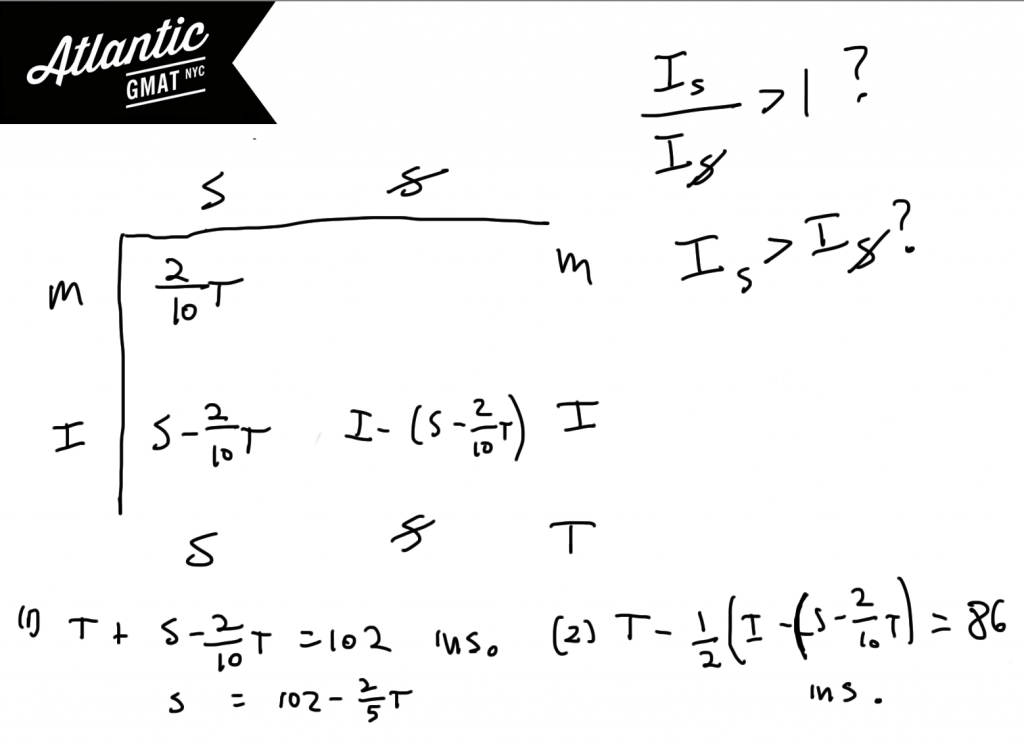# GMAT Question of the Day - Data Sufficiency - Divisibility

What is the greatest common factor of positive integers k and m?

(1) When integer k is divided by two the result is an integer with no prime factors.

(2) m = 25

# GMAT Question of the Day - Data Sufficiency - Inequalities

In a certain forest 1/5 of the trees are Walnuts and 1/6 of the trees are Birches. What is the number of birch trees in the forest?

(1) The difference between the number of Walnut trees and the number of Birch trees is greater than 10

(2) There are fewer than 350 total trees

## GMAT Question of the Day Solution

Which of the following sets has the same standard deviation as the set that contains k, k + 2, and 2k?

A. k-2, -2, 0

B. 2k, 2k + 4, 4k

C. 1, 2, k

D. k, k-1, k-2

E. k/2, k, 2

# GMAT Question of the Day - Data Sufficiency - Overlapping Sets

Of the leopards at a certain zoo, 20% are both spotted and mature. If all the leopards at the zoo are either spotted or not spotted, or mature or immature, is the ratio of the number of immature leopards who are spotted to the number of immature leopards who are not spotted greater than 1?

(1) If the number of immature spotted leopards doubled than there would be 102 total leopards at the zoo.

(2) If the number of immature but not spotted leopards were decreased by a half there would 86 total leopards at the zoo.

## GMAT Question of the Day Solution

This overlapping sets question might leave you feeling a bit empty. Thinking - what was the point of that? Well, the point of that was to define the information that you were given and then to realize that you didn't have enough to answer the questions. That's what DS questions with an "E" answer are like. Sometimes there is no further meaning than just plain old insufficient.# GMAT Question of the Day - Problem Solving - Percent

At the beginning of the year in town X, 30% of the population had liberal values. At the end of the year the total population had grown by 12% and the proportion of the population with liberal values had decreased by 5%. From the beginning of the year to the end of the year what was the approximate percent increase in the number of people in town x with non-liberal values?

A. 9%

B. 10%

C. 12%

D. 15%

E. 17%

# GMAT Question of the Day Solution

Here comes another challenging GMAT word problem. In GMAT tutoring the lesson on word problems revolves around three things:

1. Taking the time to read and process before calculating

2. Defining the question

3. Looking for ways to simplify (reduce, factor, approximate)

In this case we are looking for the percent change (a GMAT subject that comes up pretty often) of the non-liberal population. We're only given proportions so it might be helpful to pick some values. For this type of question you'll generally be picking a value for the total population from which you'll derive the rest of the values. There are some questions for which you'll pick multiple values but that should be evident from the wording of the question. In percent questions a total that is a multiple of 100 tends to work pretty well.

It is super helpful to define what you are looking for. If you fail to do so this question could become and arithmetic nightmare. If you plan well, with one tiny approximation (moving 143 up to 145) you end up with a very clean result. If you were completely stuck you could do come educated guessing on this one. You know that the population went up 12% but that the increase in non-liberals was greater than the increase in liberals. So the increase in non-liberals must have been greater than 12%. That eliminates A, B, and C.# CONTACT

Atlantic GMAT

405 East 51st St.

NY, NY 10022

(347) 669-3545

info@AtlanticGMAT.com